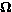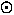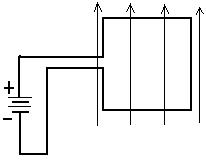## University of California, San Diego Physics 1b - Thermal Physics & Electromagnetism

 H. E. Smith Spring 2000Physics 1B - Tutorial #8The figure at the right represents the circuit of an emergency highway flasher. The flash lamp itself has a very high resistance (~107) at voltages below 110V. At voltages greater than 110V, gas inside the flasher becomes ionized and conducts electricity; the resistance is then 10. Explain how the flasher works. What determines the time between flashes? How long is it? What is the duration of the flash? Practice the right hand rule for vector cross-products; write on your fingers the appropriate vectors for Force, Velocity, B-field. If you don't wash your hands before Friday, you can use your fingers as a reference for the quiz. An electron is moving in the earth's magnetic field with velocity, v = 1.4 m/s. The particle's components of velocity are 1 m/s parallel to the field and 1 m/s perpendicular to the field, which has a magnitude of 10-4T. Describe quantitatively the motion of the particle. We sometimes say that particles, such as solar wind particles "trapped" in the earth's Van Allen Belts, are constrained to move along magnetic field lines. Use the above example to discuss the meaning of this statement. The sun has a magnetic field which is about equal in magnitude to that of the earth. In the case of the sun, field lines thread through the solar plasma, which rotates "differentially - that is the ionized gas at the equator rotates much faster than that at the poles. What do you think this does to the magnetic field? The figure below shows three configurations of a set of parallel wires. Wire 3 is always equidistant from Wires 1 and 2. The magnitudes of the currents in the wires is the same with "" indicating current directed into the page and "" indicating current out of the page. Find the direction of the net force on Wire 3 in each case. In figure (c) the distance between nearby wires is 10cm and the current is 1A in each wire. Calculate the force on Wire 3.Problem 4A Square loop of wire is placed in the magnetic field as shown. Is there a net force on the loop? If so in what direction? If not, why not? Does the loop rotate? If so, which edge rotates upward out of the page? If not, why not?Physics 1B HomeTutorials

Gene Smith